﻿ Interpolation-Collocation Method of Solution for Solving Poisson Equation

Like us on:

# Interpolation-Collocation Method of Solution for Solving Poisson Equation

Sunday Babuba*
Department of Mathematics, Federal University Dutse, Nigeria
*Corresponding Author: Sunday Babuba, Department of Mathematics, Federal University Dutse, Nigeria, Tel: 0807 079 3965, Email: [email protected]

Received Date: Oct 12, 2017 / Accepted Date: Jan 21, 2018 / Published Date: Jan 30, 2018

### Abstract

In this paper, we consider the system of algebraic equations arising from the discretization of elliptic partial differential equation with respect to x and y axes. To compute the solution of the resulting equations we use the new method to solve various elliptic equations. We study the numerical accuracy of the method. The numerical results have shown that the method provided exact result depending on the particular equation on which the scheme is applied.

Keywords: Continuous method; Lines; Multistep collocation; Elliptic; Taylor’s polynomial

#### Introduction

A finite difference scheme with continuous coefficients for the approximate solution of elliptic partial differential equation of the form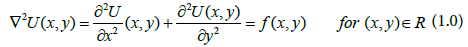And U(x,y)=G(x,y) for (x,y)∈S where R={(x,y):a<x<b, c<y<d and S enotes the boundary of R is proposed. For this discussion we assume that both f and g are continuous on their domains so that a unique solution to equation (1.0) is ensured. The method to be used is the adaptation of the canonical polynomials Qr(x,y) [1-17]. Many problems in engineering and sciences cannot be formulated in terms of partial differential equations. The vast majority of equations encountered in practice cannot, however, be solved analytically, and recourse must necessarily be made to numerical methods.

#### Our Present Method

The basic method seeks an approximation of the form: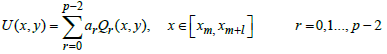(2.0)

Such that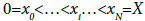The basis function,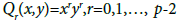are assumed known, ar are constants to be determined and pl+s, where s is the number of collocation points. The equality holds if the number of interpolation points used is equal to l. There will be flexibility in the choice of the basis function Qr(x,y) as may be desired for specific application. For this work, we consider the Taylor’s polynomial Qr(x,y)=xryr. The interpolation values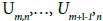are assumed to have been determined from previous steps, while the method seeks to obtain Um+l-1,n [8-27].

We apply the above interpolation conditions on eqn. (2.0) to obtain: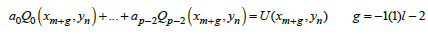(2.1)

We can write eqn. (2.1) as a simple matrix equation as,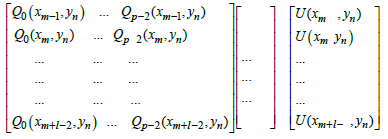(2.2)

Using three interpolation points and one collocation point, eqn. (2.1) becomes,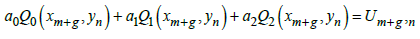(2.3)

Putting the values of g in eqn. (2.3) and writing it as a matrix we obtain,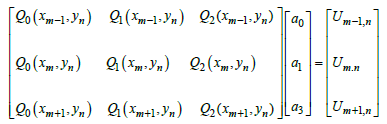(2.4)

From eqn. (2.4) we obtain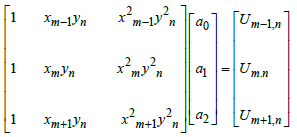(2.5)

We solve eqn. (2.5) to obtain the value of a2 as: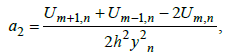Using 3 interpolation points and 1 collocation point, implies that r. Putting the values of r in eqn. (2.0) we obtain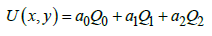(2.6)

By substitution of Q0, Q1 and Q2 in eqn. (2.6) we obtain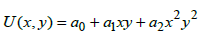(2.7)

Substituting the value of a2 in eqn. (2.7) we have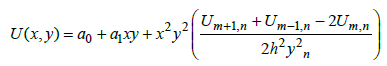(2.8)

Taken the first and second derivatives of eqn. (2.8) with respect to x we have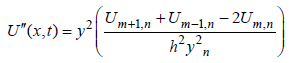(2.9)

We collocate eqn. (2.9) at y=yn, we obtain: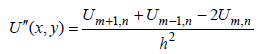(2.10)

We interchange the roles of x and y in eqn. (2.0) and applying the same interpolation conditions we obtain,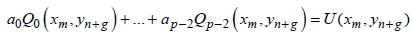(2.11)

We can write eqn. (2.11) as a simple matrix equation as,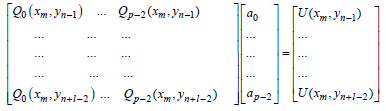(2.12)

Using 3 interpolation and 1 collocation points, eqn. (2.11) becomes,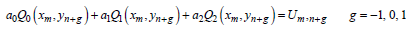(2.13)

Putting the values of g in eqn. (2.13) and writing it as a matrix we obtain,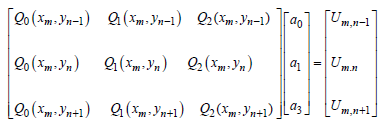(2.14)

From eqn. (2.14) we obtain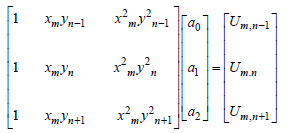(2.15)

We solve eqn. (2.15) to obtain the value of a2 as,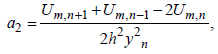Using 3 interpolation points and 1 collocation point implies that r. Putting the values of r in eqn. (2.0) we obtain,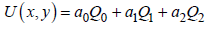(2.16)

By substitution of Q0, Q1 and Q2 in eqn. (2.16) we obtain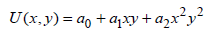(2.17)

Substituting the value of a2 in eqn. (2.17) we have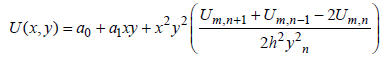(2.18)

Taken the first and second derivatives of eqn. (2.18) with respect to y we have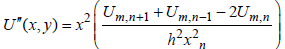(2.19)

We collocate eqn. (2.19) at x=xn to arrive at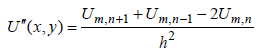(2.20)

Substituting eqns. (2.10) and (2.20) in eqn. (1.0) we obtain a scheme that solves elliptic equation. To illustrate the method we use it to solve two test problems (3.1) and (3.2) respectively.

#### Specific Problem

Example 3.1

Use the scheme to approximate the solution of a problem of determining the steady-state heat in a thin metal plate in the shape of a square with dimensions 0.5 meters by 0.5 meters, which is held at 0° Celsius on two adjacent boundaries while the heat on the other boundaries increase linearly from 0° Celsius at one corner to 100° Celsius where these sides meet. If we replace the sides with zero boundary conditions along the x- and y-axes, the problem is expressed mathematically as: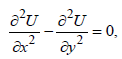for (x,y) in theR={(x,y):0<x<.5, 0<yU(0,y)=U(x,0)=0 and U(x,.5)=200x, U(.5,y)=200y

The exact solution of the problem is U(x,.y)=400xy

Using mesh size of 0.125 on each axis, the method gives us the result as shown in Table 1.

i j xi Yj Our Method Exact result
1 3 0.125 0.375 18.75 18.75
2 3 0.250 0.375 37.50 37.50
3 3 0.375 0.375 56.25 56.25
1 2 0.125 0.250 12.50 12.50
2 2 0.250 0.250 25.00 25.00
3 2 0.375 0.250 37.50 37.50
1 1 0.125 0.125 6.25 6.25
2 1 0.250 0.125 12.50 12.50
3 1 0.375 0.125 18.75 18.75

Table 1: Result of action of eqn. (2.21) on problem 3.1.

Example 3.2

Use the scheme to approximate the solution to the Poisson’s equation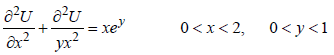With the boundary conditions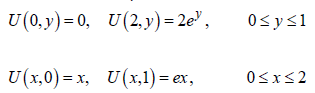The exact solution of the problem is U(x,y)=xey. Using a mesh size of 0.3333 on the axis x and 0.2000 on the y- axis we obtain the following result (Table 2).

i j xi yj Our method Exact result Error
1 1 0.3333 0.2000 0.40726 0.40713 1.30 × 10-4
1 2 0.3333 0.4000 0.49748 0.49727 2.08 × 10-4
1 3 0.3333 0.6000 0.60760 0.60737 2.23 × 10-4
1 4 0.3333 0.8000 0.74201 0.74185 1.60 × 10-4
2 1 0.6667 0.2000 0.81452 0.81472 2.55 × 10-4
2 2 0.6667 0.4000 0.99496 0.99455 4.08 × 10-4
2 3 0.6667 0.6000 1.21520 1.21470 4.37 × 10-4
2 4 0.6667 0.8000 1.48400 1.48370 3.15 × 10-4
3 1 1.0000 0.2000 1.22180 1.22140 3.64 × 10-4
3 2 1.0000 0.4000 1.49240 1.49180 5.80 × 10-4
3 3 1.0000 0.6000 1.82270 1.82210 6.24 × 10-4
3 4 1.0000 0.8000 2.22600 2.22550 4.51 × 10-4
4 1 1.3333 0.2000 1.62900 1.62850 4.27 × 10-4
4 2 1.3333 0.4000 1.98980 1.98910 6.79 × 10-4
4 3 1.3333 0.6000 2.43020 2.42950 7.35 × 10-4
4 4 1.3333 0.8000 2.96790 2.96740 5.40 × 10-4
5 1 1.6670 0.2000 2.03600 2.03570 3.71 × 10-4
5 2 1.6670 0.4000 2.48700 2.48640 5.84 × 10-4
5 3 1.6670 0.6000 3.03750 3.03690 6.41 × 10-4
5 4 1.6670 0.8000 3.70970 3.70920 4.89 × 10-4

Table 2: Result of action of eqn. (2.21) on problem 3.2.

#### Conclusion

A continuous interpolation collocation method is proposed for solving elliptic partial differential equations. To check the numerical method, it is applied to solve two (2) different test problems with known exact solutions. The scheme produced real values in test problem 1, while there is small deviation from the exact solutions in the result of the second test problem. The numerical results confirm the validity of the new numerical scheme and suggest that it is a viable numerical method which involves the reduction of PDE to a system of ODEs.

#### References

Citation: Babuba S (2018) Interpolation-Collocation Method of Solution for Solving Poisson Equation. J Biom Biostat 9: 388. DOI: 10.4172/2155-6180.1000388

Copyright: ©2018 Babuba S. This is an open-access article distributed under the terms of the Creative Commons Attribution License, which permits unrestricted use, distribution, and reproduction in any medium, provided the original author and source are credited.

Select your language of interest to view the total content in your interested language

##### Recommended Journals
Viewmore
###### Article Usage
• Total views: 3296
• [From(publication date): 0-2018 - Dec 07, 2019]
• Breakdown by view type
• HTML page views: 3190Can't read the image? click here to refresh